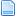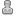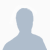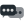Hello Guest it is August 17, 2022, 01:31:19 PM

###Author Topic: need help!  (Read 2131 times)

0 Members and 1 Guest are viewing this topic.

####Oldraven•30##### need help!
« on: February 20, 2015, 05:36:49 AM »
Hello all,

I really could use some help with one command in the VB script below.

This statement works but also seeds the X- and Z-axes DRO's with random digits!
Call SetOEMDRO(824,number)                  'Set tool # in DRO
Why ??
I am working on this for almost a week now.
For the rest the script works OK.

Jos

'REM _________Script to advance ATC/Turret 1 position.____________

maxtool = 6                        '# of tools in turret
extraAngle = 6                             'Extra angle added to turret rotation
round = 360                        'One turn in degrees of turret
oldtool = getcurrenttool                       'Tool # before rotation
newtool = GetOEMDRO(824)               'Get Tool #
Angle = round/maxtool                                     'Angle of rotation i.e. 60 degrees
number = GetOEMDro(824)                                 'Get Tool #

Code "G91"                        'Incremental Distance Mode
If GetOEMDRO(824) = 0 Or GetOEMDRO(824) >6 Then
number1 = Question("What is current tool # ?")
Call SetOEMDRO(824,number1)
Call SetOemDro(803,number1*round/maxtool) '803 = A Turret DRO
Exit Sub
End If
number=number+1                                            'add 1 to the #
If nummer>maxtool Then number=1                        'check if tool # is 6

'After executing the next line the X- and Z- DRO's display random figures.
'I do not know why.
Call SetOEMDRO(824,number)                                 'Set tool # in DRO

'now calculate the rotation angle of turret
If number=1 Then Call SetOemDro(803,0)
ToolChange = GetOEMDRO(803)                    'Get A position
ToolChange = (round/maxtool)+extraAngle                  'Tool-change turret 60 + 6 CW

Code "G0 A" & ToolChange                                'execute rotation CW
code "G0 A" & -6                          '6 steps CCW

While IsMoving()
Wend
Call SetOEMDro(803,((number-1)*round/maxtool))

Code "G90"                             'back to Absolute Distance Mode
« Last Edit: February 20, 2015, 05:43:47 AM by Oldraven »

####BR549•6,965##### Re: need help!
« Reply #1 on: February 20, 2015, 12:55:49 PM »
Yo cannot use the word ROUND as a variable it is an internal math function. Change that word to something else. I changed it to Turn.

maxtool = 6                        '# of tools in turret
extraAngle = 6                             'Extra angle added to turret rotation
Turn = 360                        'One turn in degrees of turret
oldtool = getcurrenttool                       'Tool # before rotation
newtool = GetOEMDRO(824)               'Get Tool #
Angle = Turn/maxtool                                     'Angle of rotation i.e. 60 degrees
number = GetOEMDro(824)                                 'Get Tool #

Code "G91"                        'Incremental Distance Mode
If GetOEMDRO(824) = 0 Or GetOEMDRO(824) >6 Then
number1 = Question("What is current tool # ?")
Call SetOEMDRO(824,number1)
Call SetOemDro(803,number1*Turn/maxtool) '803 = A Turret DRO
Exit Sub
End If
number=number+1                                            'add 1 to the #
If nummer>maxtool Then number=1                        'check if tool # is 6

'After executing the next line the X- and Z- DRO's display random figures.
'I do not know why.
Call SetOEMDRO(824,number)                                 'Set tool # in DRO

'now calculate the rotation angle of turret
If number=1 Then Call SetOemDro(803,0)
ToolChange = GetOEMDRO(803)                    'Get A position
ToolChange = (Turn/maxtool)+extraAngle                  'Tool-change turret 60 + 6 CW

Code "G0 A" & ToolChange                                'execute rotation CW
code "G0 A" & -6                          '6 steps CCW

While IsMoving()
Wend
Call SetOEMDro(803,((number-1)*Turn/maxtool))

Code "G90"                             'back to Absolute Distance Mode

####Oldraven•30##### Re: need help! SOLVED!
« Reply #2 on: February 21, 2015, 04:15:16 AM »

You cannot use the word ROUND as a variable it is an internal math function. Change that word to something else. I changed it to Turn.

Thanks for this hint.

I am Dutch, hence the mistake in translating the dutch word "rond"to "round".
I did not know that Round is an internal function.

However, the strange problem seems to be solved.

I decided to create a new profile, from the one that gave me so much trouble, in order to work on this new profile with Machscreen.

Before doing any changes to to lset file I tested the new profile.
After copying the M6Start.m1s file in the profile folder all worked like a charm.

The X- and Z DRO's stay on zero!! no more jumping around at random.

It seems that copying and thus creating a new profile Mach3 corrected a mistake somewhere.

Regards,

Jos